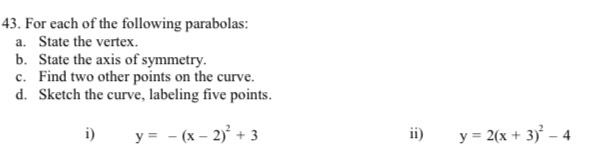### ¿Todavía tienes preguntas de matemáticas?

Pregunte a nuestros tutores expertos
Algebra
Pregunta43. For each of the following parabolas: a. State the vertex. b. State the axis of symmetry. c. Find two other points on the curve.

i) $$y = - ( x - 2 ) ^ { 2 } + 3$$

ii) $$y = 2 ( x + 3 ) ^ { 2 } - 4$$

(i)

Vertex (2,3)

Axis of symmetry: x=2

two points on the graph: (4,-1) and (3,2)

(ii)

Vertex (-3,-4)

Axis of symmetry: x=-3

two points on the graph: (-5,4) and (-4,-2)

Solución
View full explanation on CameraMath App.